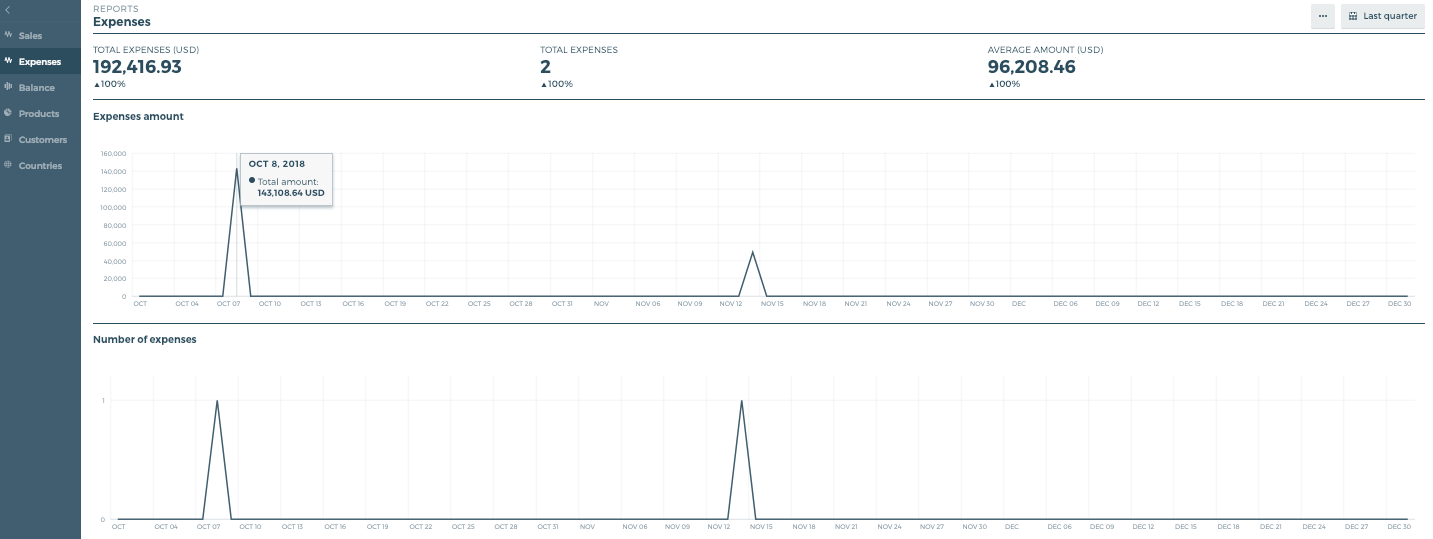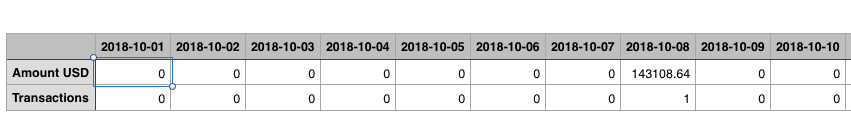# Expenses report

The expenses report gives you an overview of your expenses. All amounts include tax (gross amounts).• Total expenses (currency) - Your total expenses. The total amount registered in your Quaderno account as expenses for the given period.
• Total expenses - The total number of expenses registered in your Quaderno account for the given period.
• Average amount - The average amount of an expense.
• Expenses amount - The total sum of expenses on a given day.
• Number of expenses - The number of expenses on a given day.